# Know all about Switch Mode Power Supply

A switch mode power supply (SMPS) is basically an electronic power supply which efficiently converts electrical power. It transforms power coming from the main source and gives it to the load like transformer. It converts current and voltage characteristics. The transistor in this supply switches between low dissipation, full off and full on states and therefore it does not spend much time in the high transition states. Due to this, the wastage of energy is minimized. By changing the on and off time we achieve voltage regulation in it. These switch mode power supplies are usually smaller in size and lighter in weight as compared to the linear supply.  This is mainly because of the smaller size and weight of the transformer used in it.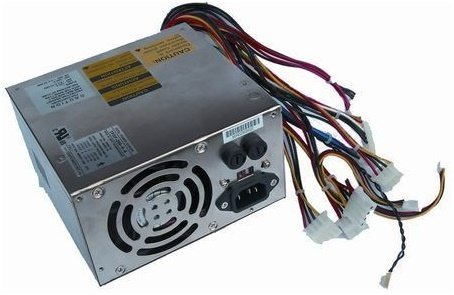### Switch Mode Power Supply

The switching regulators are mostly used where we require highly efficient and smaller regulators. But their simpler designs can have a poor power factor, noise problems and they are also more complicated. SMPS have many applications in power electronics projects.

### TOPOLOGIES OF SMPS

SMPS has different topologies in it where there are different specifications and features of every topology. In order to select the most suited topology, the complexity, drawbacks, basic operation, advantages and area of usage should be known. The following features must be kept in mind while choosing a topology:

1. What is the range of the output voltage as compared to the input voltage?
2. Number of outputs that are required?
3. Is there any need of dielectric isolation between the input and the output?
4. What is the value of input or output voltage? Is it high?
5. What is the value of input or output current? Is it high?
6. What is the maximum primary voltage across the transformer and the maximum duty cycle?

There are several topologies as mentioned below:

### WORKING OF THE DIFFERENT SMPS TOPOLOGIES

Some of the topologies and their working are described below:

#### BUCK SMPS

It is one of the most common and simplest topologies. It is ideally used as a DC to DC converter to step down the DC voltage. High efficiency and high power levels can be achieved through it. But a disadvantage of this converter is that the input current is discontinuous. For single phase applications a single inductor is required.The Buck converter is also called as a ‘chopper circuit’. The figure below shows the circuit diagram as well as the waveforms of current and voltages.The output voltage is taken across the load resistor RL. Its frequency is f=1/T.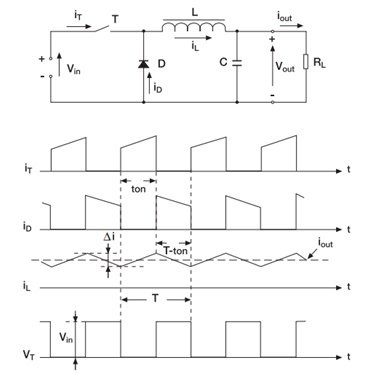In this converter, a switch is placed at the start of the circuit and in series with the input source. When the switch is on, during the period TON, the energy goes to the output and to the inductor. The value of the current flowing through the inductor increases. When the switch is off, the current stored in the inductor continues to flow during the period Toff. This current passes through the diode which is then called as a freewheeling diode. During Toff, reverse voltage is applied across the inductor and the value of current therefore decreases as shown in the waveforms.The relationship between the input and output is shown by the equation:

Vout= D.Vin

Where D= duty cycle.

#### BOOST SMPS

This topology is also non-isolating. The buck steps down the voltage whereas the boost steps up the voltage. The voltage at the output is greater than the voltage at the input. In the continuous conduction mode, it draws current in the continuous manner therefore it is best suited for power factor correction circuits.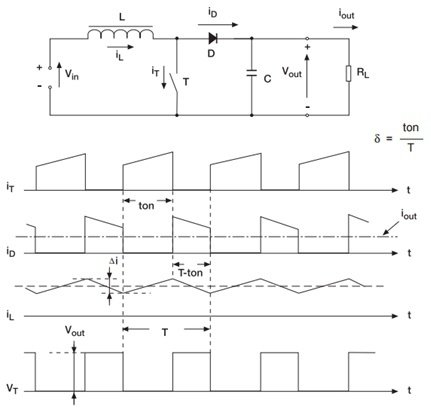In a boost converter, an inductor is placed at the start of the circuit in series with the input voltage source. During Ton, when the switch is opened, the energy is given to the inductor by the input source. The value of the current flowing through the inductor increases. During the on time, the output capacitor supplies the output current to the load. When the switch is off, during the period of Toff, the energy is applied to the load from the source. And the current through the inductor continues to flow but the value of this current now starts decreasing as shown in the waveforms.The relationship between the input and output is shown by the equation:

Vout= Vin/(1-D)

Where D=duty cycle.

#### BUCK-BOOST SMPS

The buck boost topology can either step up or step down the voltage according to the requirements. But it has a few disadvantages such as the inverting of output voltage and it also lacks a ground which makes the driver circuit very complicated.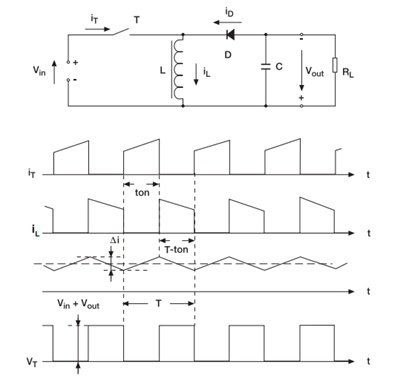In the buck boost converter , the components of both the buck and the boost are combined and a control circuit is added which detects the level of voltages and then acts accordingly.

#### FLYBACK SMPS

This topology is isolated by using a transformer in the circuit. The circuit is similar to the buck boost circuit. As a transformer is used therefore the different levels of voltages can be achieved. Mostly it is used for low power applications. The peak currents can be very high but the output current should not exceed beyond 10A.One main advantage of this topology is that there is no need of a separate inductor, the transformer itself behaves as an inductor. It has a simple circuitry which makes it cost effective and easy to use.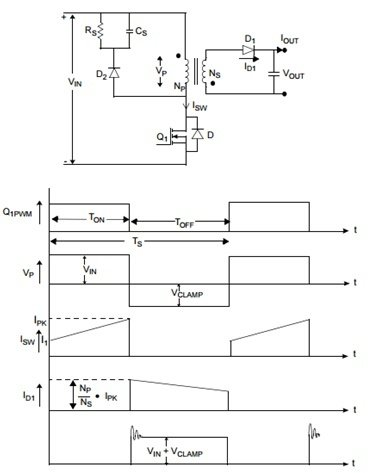In this converter, a switch is added with the primary of the transformer. The transformer provides isolation between the input and output sources. During the Ton period, when the switch is on, the dot side acts as a positive and the diode is reverse biased due to which the transformer becomes an inductor and stores energy. The value of current in the primary winding of the transformer increases. During this time, the capacitor supplies output current to the load.

When the switch is turned off, negative voltage is induced by the magnetizing current on the dot side. The diode is then forward biased and the secondary voltage of the transformer becomes equal to the output voltage. The stored energy in the primary side transfers to the secondary by the flyback action. It charges the capacitor on the output and also gives energy to the load connected.

PUSH PULL CONVERTERS:

The push pull converters contain a set of two primary windings. The transformer core is utilized very efficiently in this topology but there are significant copper losses. Their main advantage is that high powers can be achieved through it as compared to the flyback converter. The switching can be difficult because we cannot turn on both the switches at the same time. If the switches are turned on at the same time then a high surge current will destroy the circuit.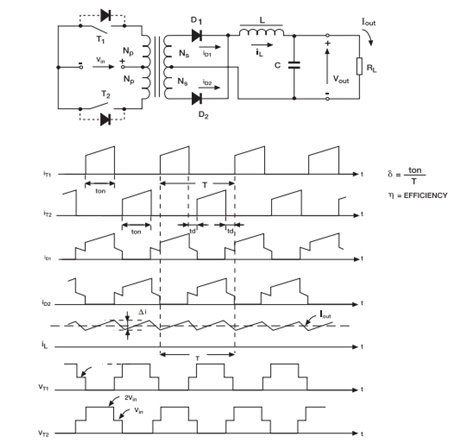A center tapped transformer is used in it, the primary and the secondary, both are center tapped. Two switches are connected with the primary winding, one at each end. These switches operate during alternate half cycles. Pulsating voltage is produced at the primary of the transformer. The switches are controlled by a control unit or a driver circuit.

ADVANTAGES OF SMPS OVER LINEAR POWER SUPPLY:

1. The frequency of SMPS is very high therefore the transformer used in it is smaller as compared to the transformer used in the linear power supply.
2. Switching mode supply is also lighter in weight.
3. They are more efficient as compared to linear power supply because of no significant heat dissipation or copper losses.
4. The variation of voltage is very less when load is added.
5. It has a higher power factor.
6. Their noise is in the inaudible human range.

### comparison between linear and switch mode power supplies

The table above summarizes the comparison between linear and switch mode power supplies: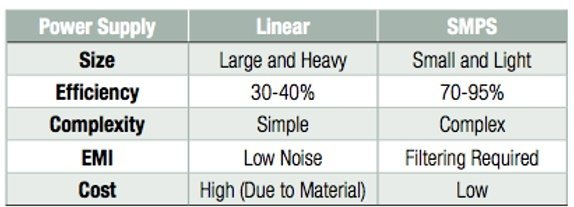### Application based project using SMPS

Following are the SMPS based project for final year engineering students: